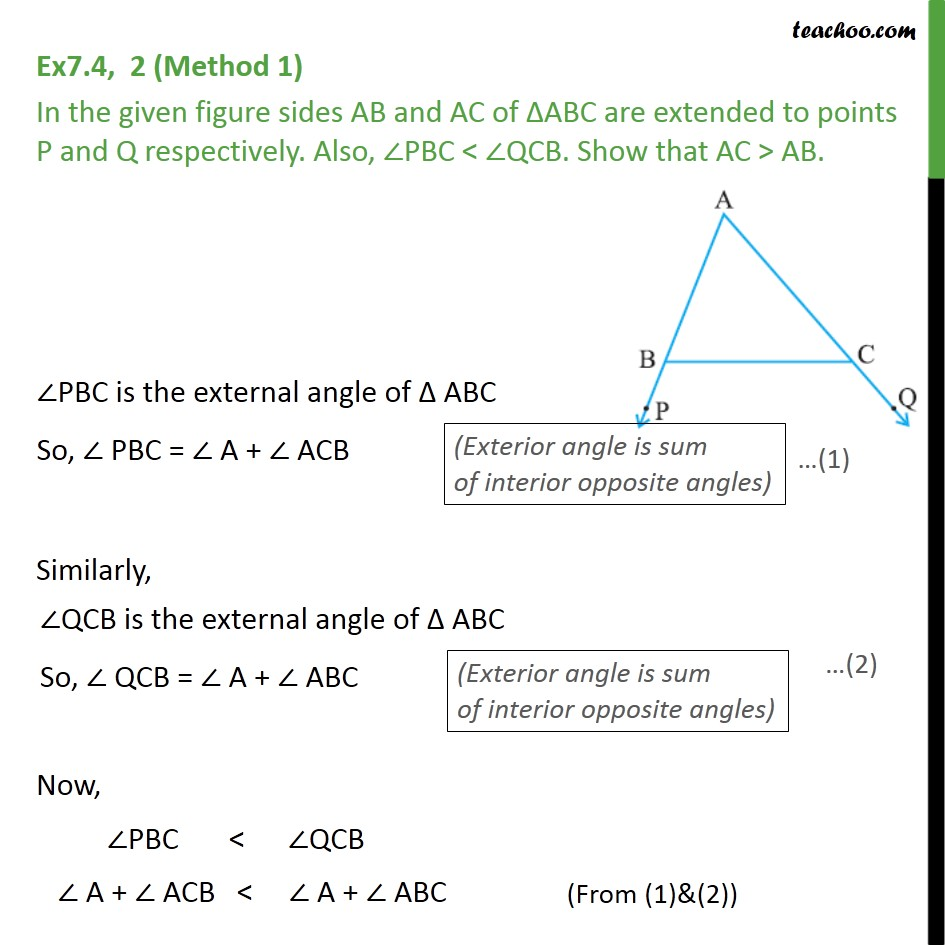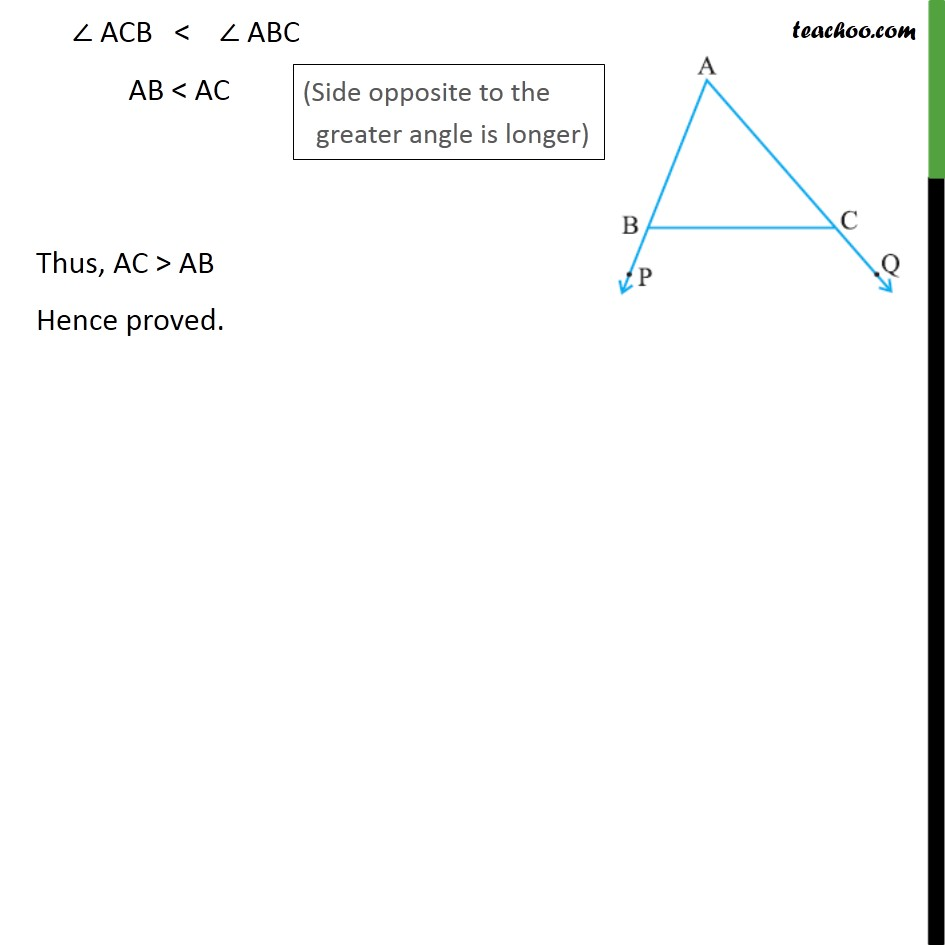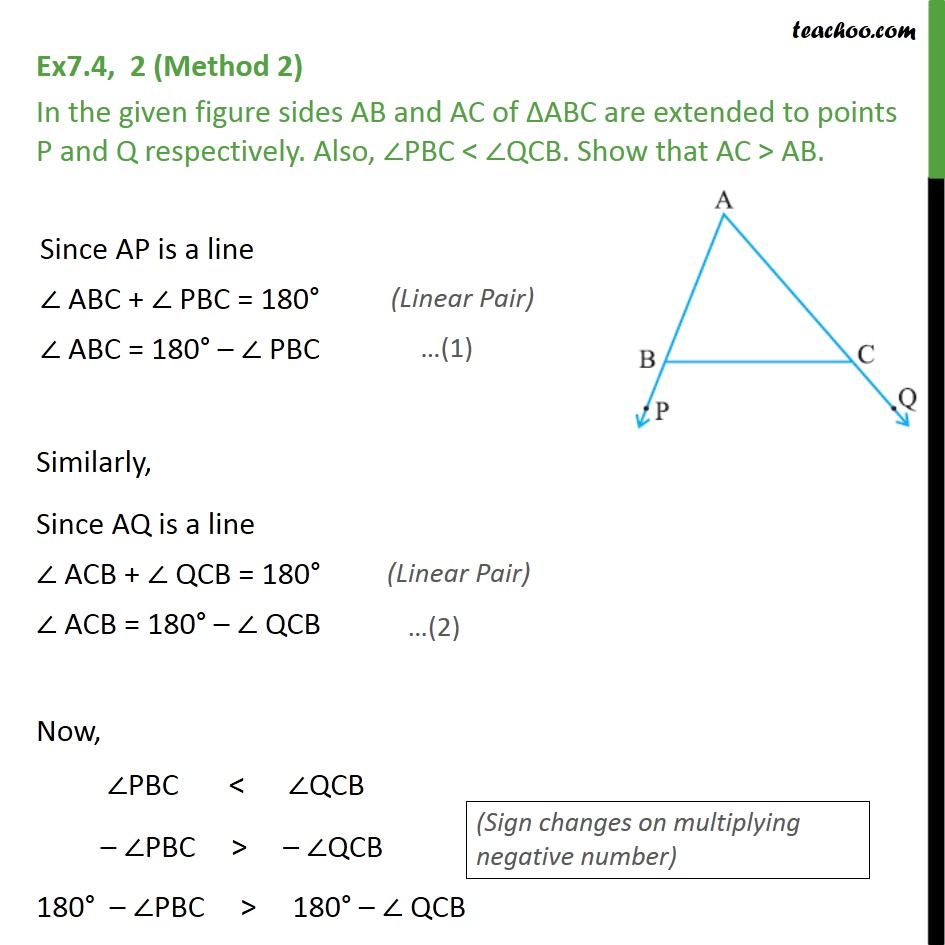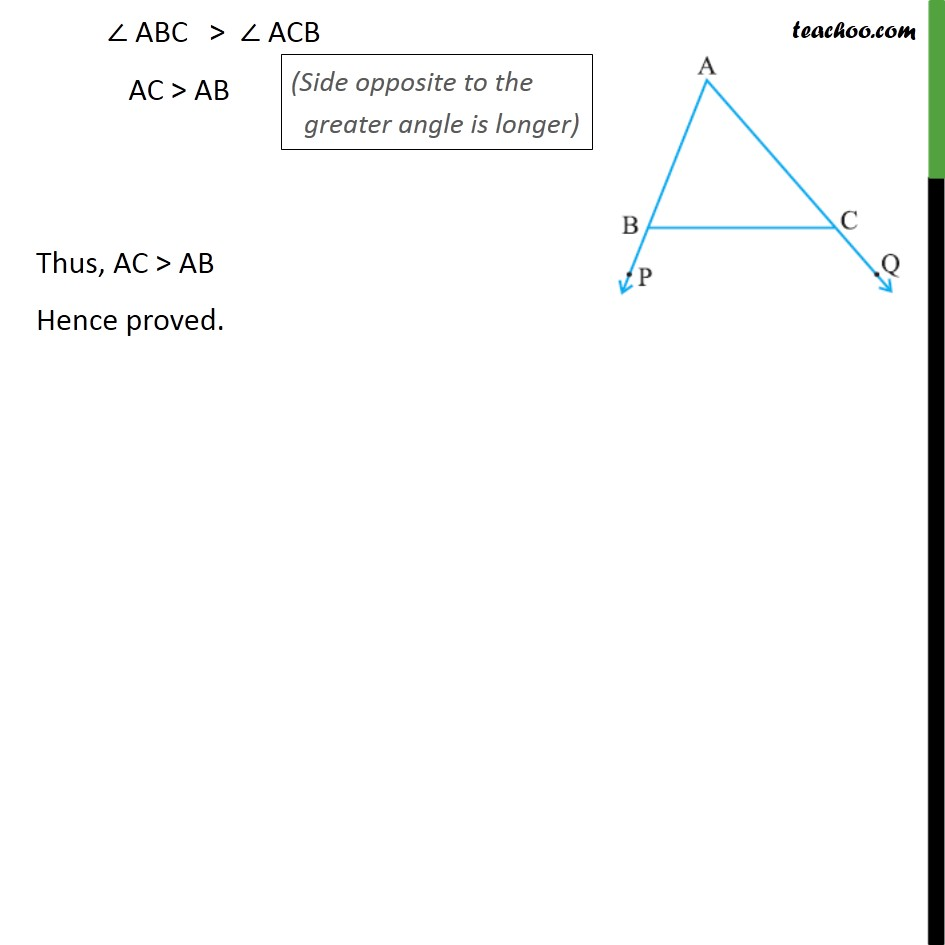1. Chapter 7 Class 9 Triangles
2. Serial order wise
3. Ex 7.4

Transcript

Ex7.4, 2 (Method 1) In the given figure sides AB and AC of ABC are extended to points P and Q respectively. Also, PBC < QCB. Show that AC > AB. Similarly, Now, PBC < QCB A + ACB < A + ABC ACB < ABC AB < AC Thus, AC > AB Hence proved. Ex7.4, 2 (Method 2) In the given figure sides AB and AC of ABC are extended to points P and Q respectively. Also, PBC < QCB. Show that AC > AB. Similarly, Now, PBC < QCB PBC > QCB 180 PBC > 180 QCB

Ex 7.4

Chapter 7 Class 9 Triangles
Serial order wise